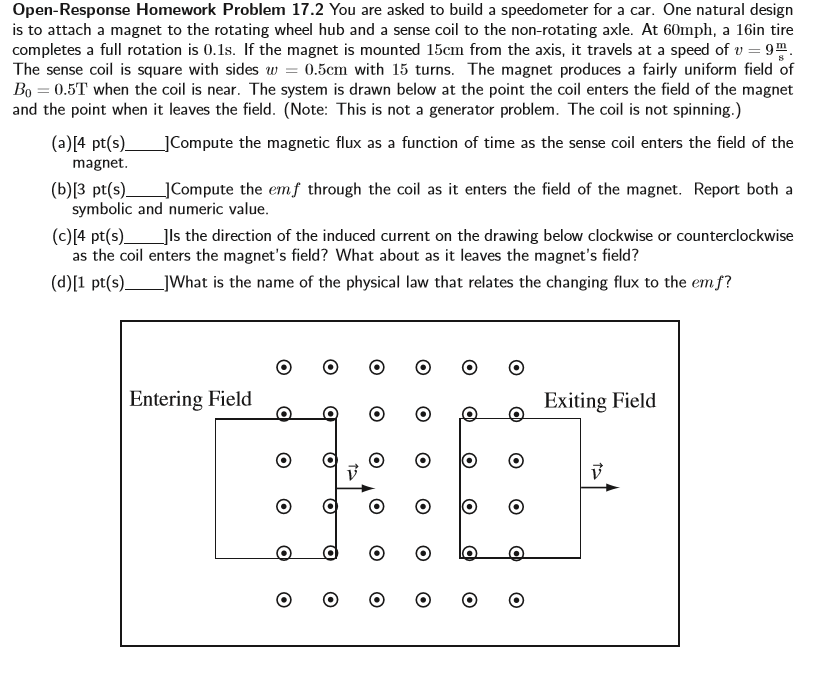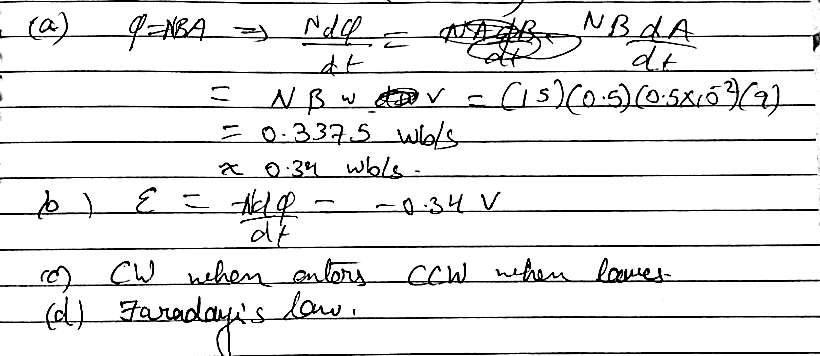Homework Help Question & Answers

# You are asked to build a speedometer for a car. One natural design is to attach...You are asked to build a speedometer for a car. One natural design is to attach a magnet to the rotating wheel hub and a sense coil to the non-rotating axle. At 60mph, a 16in tire completes a full rotation is 0.1s. If the magnet is mounted 15cm from the axis, it travels at a speed of upsilon = 9 m/s. The sense coil is square with sides w = 0.5cm with 15 turns. The magnet produces a fairly uniform field of B_0 = 0.5T when the coil is near. The system is drawn below at the point the coil enters the field of the magnet and the point when it leaves the field. (a) Compute the magnetic flux as a function of time as the sense coil enters the field of the magnet. (b) Compute the emf through the coil as it enters the field of the magnet. Report both a symbolic and numeric value. (c) Is the direction of the induced current on the drawing below clockwise or counterclockwise as the coil enters the magnet's field? What about as it leaves the magnet's field? (d) What is the name of the physical law that relates the changing flux to the emf?

#### Homework Answers

Answer #1Know the answer?
Your Answer:

#### Post as a guest

Your Name:

What's your source?

#### Earn Coin

Coins can be redeemed for fabulous gifts.

Not the answer you're looking for? Ask your own homework help question. Our experts will answer your question WITHIN MINUTES for Free.
Similar Homework Help Questions